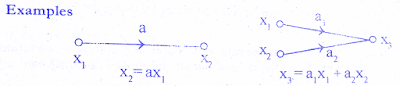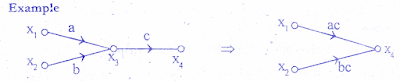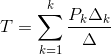# Signal Flow Graph of Control System

0
151

## Explanation of terms used in Signal Flow Graph:

#### Node : A node is a point representing a variable or signal.

Branch : A branch is directed line segment joining two nodes. The arrow on the branch indicates the direction of signal flow an the gain of a branch. is the transmittance.
Transmittance : The gain acquired by the signal when it travels from one node to another is called transmittance.The transmittance can he real or complex.
Input node ( Source) : It is a node that has only outgoing branches.

Output node ( Sink ) : It is a node that has only incoming branches.

### Signal Flow Graph Algebra:

#### Rule 1 : Incoming signal to a node through a branch is given by the product of a signal at previous node and the gain of the branch.Check below solved examples.#### Rule 2 : Cascaded branches can be combined to give a single branch whose transmittance is equal to the product of individual branch transmittance.Check below solved examples.#### Rule 3 : Parallel branches may be represented by single branch whose transmittance is the sum of individual branch transmittance.Check below solved examples.#### Ruled 4 : A mixed node can be eliminated by multiplying the transmittance of outgoing branch (from the mixed node) to the transmittance of all incoming branches to the mixed node.Check below solved examples.#### Rule 5 : A loop may be eliminated by writing equations at the input and output node and rearranging the equations to find the ratio of output to input.This ratio gives the gain of resultant branch.Check below solved examples.## Signal Flow Graph Reduction:

### Mason’s Gain Formula:

#### Mason’s Gain formula states the overall gain of the system [transfer function] as follows,### Procedure for converting block diagram to signal flow graph:

#### 5. Draw the feedback paths between various nodes and mark the gain of feedback paths along with sign.

READ HERE  Registers & Shift Registers: Definition, Function & Examples